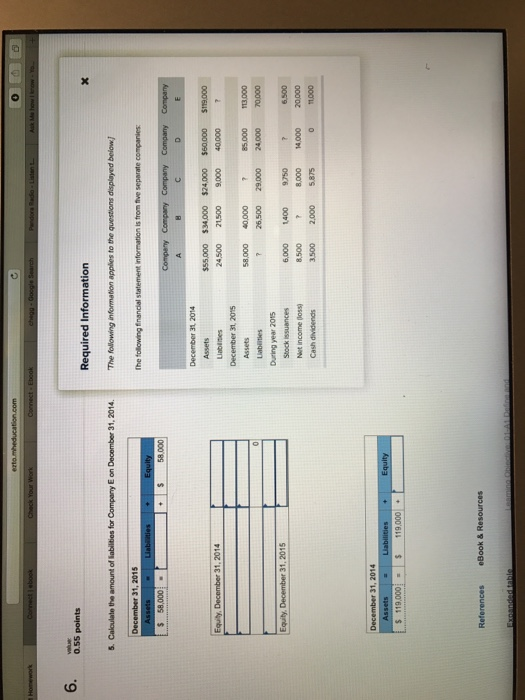# Answered Essay: Calculate the amount of liabilities for Company E on December 31, 2014. The follCalculate the amount of liabilities for Company E on December 31, 2014. The following information applies to the questions displayed below: The following financial statement information is from the separate companies:

Company A:

Total Assets = Total Liabilities + Total Equity

December 31, 2014:
\$55,000 = \$24,500 + Total Equity
Total Equity = \$30,500

December 31, 2015:
Total Equity, December 31, 2015 = Total Equity, December 31, 2014 + Stock issuances + Net income- Cash Dividends
Total Equity, December 31, 2015 = \$30,500 + \$6,000 + \$8,500 – \$3,500
Total Equity, December 31, 2015 = \$41,500

Total Assets = Total Liabilities + Total Equity
\$58,000 = Total Liabilities + \$41,500
Total Liabilities = \$16,500

Company B:

Total Assets = Total Liabilities + Total Equity

December 31, 2014:
\$34,000 = \$21,500 + Total Equity
Total Equity = \$12,500

December 31, 2015:
Total Assets = Total Liabilities + Total Equity
\$40,000 = \$26,500 + Total Equity
Total Equity = \$13,500

Total Equity, December 31, 2015 = Total Equity, December 31, 2014 + Stock issuances + Net income- Cash Dividends
\$13,500 = \$12,500 + \$1,400 + Net Income (Loss) – \$2,000
Net Income (Loss) = \$1,600

Company C:

Total Assets = Total Liabilities + Total Equity

December 31, 2014:
\$24,000 = \$9,000 + Total Equity
Total Equity = \$15,000

December 31, 2015:
Total Equity, December 31, 2015 = Total Equity, December 31, 2014 + Stock issuances + Net income- Cash Dividends
Total Equity, December 31, 2015 = \$15,000 + \$9,750 + \$8,000 – \$5,875
Total Equity, December 31, 2015 = \$26,875

Total Assets = Total Liabilities + Total Equity
Total Assets = \$29,000 + \$26,875
Total Assets = \$55,875

Company D:

Total Assets = Total Liabilities + Total Equity

December 31, 2014:
\$60,000 = \$40,000 + Total Equity
Total Equity = \$20,000

December 31, 2015:
Total Assets = Total Liabilities + Total Equity
\$85,000 = \$24,000 + Total Equity
Total Equity = \$61,000

Total Equity, December 31, 2015 = Total Equity, December 31, 2014 + Stock issuances + Net income- Cash Dividends
\$61,000 = \$20,000 + Stock Issuance + \$14,000 – \$0
Stock Issuance = \$27,000

Company E:

Total Assets = Total Liabilities + Total Equity

December 31, 2015:
\$113,000 = \$70,000 + Total Equity
Total Equity = \$43,000

December 31, 2014:
Total Equity, December 31, 2015 = Total Equity, December 31, 2014 + Stock issuances + Net income- Cash Dividends
\$43,000 = Total Equity, December 31, 2014 + \$6,500 + \$20,000 – \$11,000
Total Equity, December 31, 2014 = \$27,500

Total Assets = Total Liabilities + Total Equity
\$119,000 = Total Liabilities + \$27,500
Total Liabilities = \$91,500Pages (550 words)
Approximate price: -

Help Me Write My Essay - Reasons:Best Online Essay Writing Service

We strive to give our customers the best online essay writing experience. We Make sure essays are submitted on time and all the instructions are followed.Our Writers are Experienced and Professional

Our essay writing service is founded on professional writers who are on stand by to help you any time.Free Revision Fo all Essays

Sometimes you may require our writers to add on a point to make your essay as customised as possible, we will give you unlimited times to do this. And we will do it for free.Timely Essay(s)

We understand the frustrations that comes with late essays and our writers are extra careful to not violate this term. Our support team is always engauging our writers to help you have your essay ahead of time.Customised Essays &100% Confidential

Our Online writing Service has zero torelance for plagiarised papers. We have plagiarism checking tool that generate plagiarism reports just to make sure you are satisfied.Try it now!

## Calculate the price of your order

Total price:
\$0.00

How it works?Fill in the order form and provide all details of your assignment.Proceed with the payment

Choose the payment system that suits you most.HOW OUR ONLINE ESSAY WRITING SERVICE WORKS

Let us write that nagging essay.By clicking on the "PLACE ORDER" button, tell us your requires. Be precise for an accurate customised essay. You may also upload any reading materials where applicable.Pick A & Writer

Our ordering form will provide you with a list of writers and their feedbacks. At step 2, its time select a writer. Our online agents are on stand by to help you just in case.Editing (OUR PART)

At this stage, our editor will go through your essay and make sure your writer did meet all the instructions.